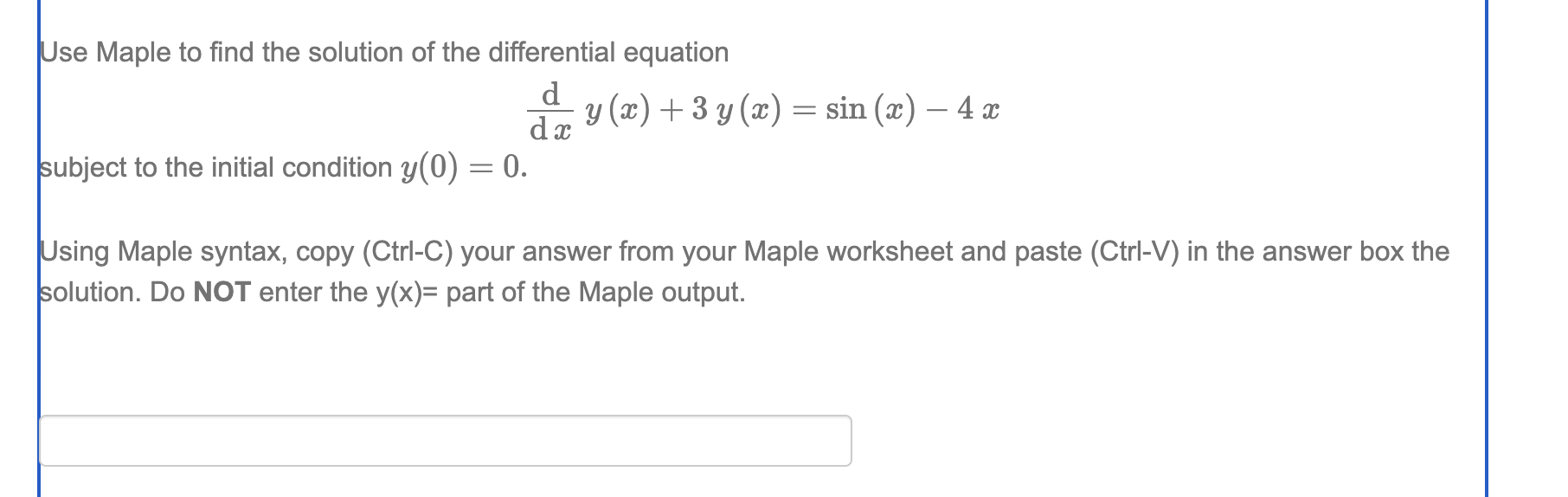## how do I solve this one?...## Calculating deviation with derivatives ...

Hello,

Ive followed the examples, and yet not the result the book gave. The question before this one, same type, and i did get the right answer there.

Would you have a clue why it is not going as planned?

Translation: "Through a resistancewire with the measured resistance R= (1.5+-0.5)Ohm goes an electric current with the measured strength I= (2.5+-0.05)A, During a timespan of t=(10+-0.5)s develops an amount of heat Q=RI^2*t. Give the estimate of the maximum relative deviation in Q."

Here i got the right answer of .42, and yet when doing the exact same thing in assignment 4, i got the wrong answers.

Translation: "Two bodies with mass M and m have a massmidpointdistance r. For the gravitational force between these bodies counts: F=y*M*m/r^2, at which y is the gravitational constant. Give an estimation of the maximum realative deviation in F on ground of the following measurementdata: M=(7.0+-0.05)kg, m=(2.0+-0.02)kg and r=(5.0)+-0.05)m."

I had the answer -0.0029. The books says its wrong, but i cant see what i did wrong.

Translation of the example: "According to the law of Ohm the currentstrength in the stream(/current)circle(circuit) with tension(voltage) U and resistance R is equal to I=U/R. For a given currentcircle((part of a)circuit) is given: U=(30.0+-0.05)Volt and R=(2.5+-0.03)Ohm. Determine the maximum absolute and relative deviation in I.

Solution

The maximum absolute deviation in I is

This gives the currentstrength:

The maximum realtive deviation in I is: .... or about 1.4%"

Could somebody tell me what i am doing wrong?

Thank you!

Greetings,

The Function> #opdracht 3
 >(1)
 >>>(2)
 >(3)
 >(4)
 >(5)
 >>(6)
 >(7)
 >(8)
 >(9)
 > #Opdracht 4
 >>(10)
 >>(11)
 >(12)
 >(13)
 >(14)
 >(15)
 >(16)
 >(17)
 >## The book didnt even gave the right answer...

The answers from the book dont make any sense after using their solution. Its not producing the expected results.

This is the translation by the way: "A battery that has a voltage U, is in series with a resistor R, and a condenser with capacity C. The current strength on a given time "t" in this circuit is given by i=i(t)=U/R*e^-(t/R*C). Determine R and C based on the data given in table 5.6, the current is U=100V."

Greetings,

The Function>